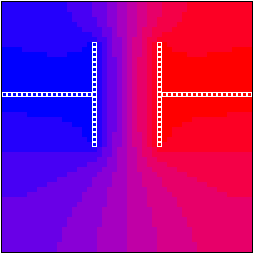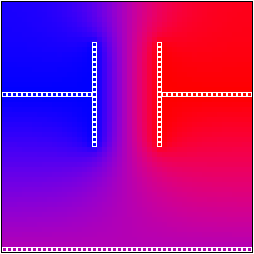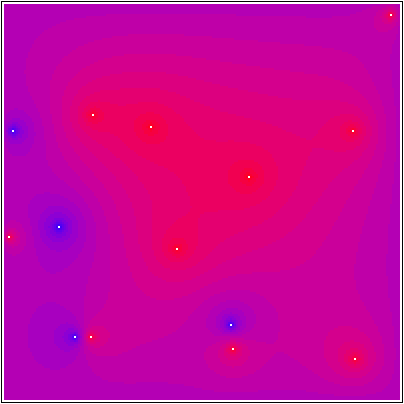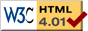Nav: [home] > [sim] > [elstat]

## Electrostatic Potential Solver

### Some theory: the Laplace equation

Imagine there is a 2dim area and you bring some fixed charges (of finite size) in there. As a consequence, a non-constant electrostatic potential will establish. In the empty parts, this potential follows the Laplace equation with the boundary conditions imposed by the fixed charges.

The Laplace equation (Laplace phi(x,y)=0 whith phi(x,y) being the potential at position (x,y)) is a partial differential equation (PDE). Numerical solving of PDEs is not as simple as solving ODEs. In order to solve the Laplace equation, this program divides up the area into little elements (discretisation of the problem; finite element method).

The second partial derivation in the Laplace equation is computed numerically using standard difference quotient of a cell with its 4 neighbour elements. This results in a quite large but sparse linear system (number of rows and columns of the Matrix is the number of floating (non-fixed) elements in the area and hence at least several thousand). In order to solve this system, iterative the following methods are implemented:

• Gauss-Seidel algorithm: This is the standard iterative algorithm for solving sparse diagonally-dominant linear systems.
• Thomas-TDMA (tridiagonal matrix algorithm): In contrast to Gauss-Seidel, this algorithm computes whole lines of successive floating elements exactly and iterates over these line chunks.

Successive overrelaxation is implemented for both these algorithms which can greatly improve the speed of convergence when a suitable factor is entered manually (see "omega" setting). The Thomas-TDMA will normally converge faster than the Gauss-Seidel algorithm but it does not allow for periodic boundary conditions (since this would destroy the tridiagonal structure of the system).

### Let's have a look

This is a screen shot of the elstat progrgram. The elements with a white border are fixed charges at a potential of +100 (red) and 0 (blue); the other elements are floating (i.e. computed numerically). You obviously instantly recognize the shown equation...### Using of the program

Okay, so if you think all that GUI-stuff is self-explaining and just want to get on with it, just note the following: Keys '+' and '-' can be used to zoom in and out and dragging with the right mouse button pressed will pan the simulation area.

Description of the GUI elements:

• Solver: allows you to select the solver to use; see above.
• Timeslice: Time in msec the solver may run until processing user events (like adding charges with the mouse) are checked again.
Note that the solver can only be interrupted after complete iterations and for large area sizes, these may take longer than the specified time slice.
• Epsilon: The two spin boxes allow you to set the PDE solver convergence threshold epsilon. In the screen shot above, "1" and "-6" means epsilon=1e-6.
The PDE solver will stop iterations when the largest change of a potential value during the current iteration is smaller than epsilon.
• Omega: This is the overrelaxation factor which is in range 1..2 (hence 100%..200%). A value of 100% (displayed as "off") switches off this feature and results in normal solver iterations. Values larger than 1 result in successive overrelaxation which will, when a proper value is set, speed up solver convergence considerably. (Watch the dots in the terminal for that.)
• Shuffle: Normal Gauss-Seidel or TDMA will iterate through the linear equation system always in the same way (i.e. top-down). When setting "shuffle" to something else than "[none]", the solvers will run lines top-down and bottom up and use linear systems generated via X and Y sweeps.
Note: Experiments showed that this will often slow down convergence a bit.
• MaxIter: Limit iterations of the solver. Forget it and leave it at "[no limit]". This is only useful for testing.
• Periodic BC: Whether to use periodiv boundary conditions (i.e. torus-like area) or not.
Note that the TDMA solver cannot handle periodic BC and hence this check box is enabled only for the Gauss-Seidel solver.
• Start/Stop: Start and stop the PDE solver. When stopped, the button displays "Start" and the PDE solver will not do anything. When running (the button displays "Stop"), the PDE solver will start running whenever something changes and will stop temporarily when the algorithm has converged. It will, however, re-start automatically once one changes some charge etc.
• Area... openes the area size dialog to change the number of elements in the area.
• Reset will fill the complete area with fixed or floating (depending what you specified as "fill type" in the area dialog; default is floating) elements of potential 0.
• Zero will set the potential value of all floating (non-fixed) elements to 0.
• NColors allows you to change the number of colors in the display. The color display will update automatically so that the lowest and highest potential value fit into color range. It is, however, sometimes interesting to limit the number of colors to "see more". See below for an example image.
• Shape: Selects the shape you can draw with the left mouse button.
• Type: Selects the type of mouzse-drawn elements.
• Potential: Choose electrostatic potential for elements drawn with the mouse.

### Examples

Here are some example potential distributions calculated with this program. (These are just cut-out screen shots.)
The number of colors in the left column was reduced to 12 so that one can see the potential isolines more easily.This is a simple planar capacitor where the right side has the potential +100 and the left side has -100. When starting at the center and walking out of the capacitor, one can see the increasing inhomogentiy of the electrical field. (The electrical field is the gradient of the displayed potential.)This is the same image as above but this time a plate with potential 0 was added at the ground (bottom side of the image). Note that the potential isolines on the bottom now run quite differently which can very well be seen in the 12-color image.Now let's put the capacitor into a sphere with ground potential (i.e. 0)... Of course, the sphere shields off the electrical field as expected.And finally, let's remove the plates of the capacitor so that only the wires stay there. (The assymetry is due to the fact that the right horizontal wire is one element above the left one.)Of course, one is not limited to the 50x50 element grid as used in the images above. On the left you can see an image of a couple of "point changes" with potential +100 (red) or -100 (blue) inside a box with potential 0 at the border, calculated on a 200x200 element grid. The number of colors was set to 25.